Function Repository Resource:

# PiecewiseD

The derivative of a piecewise function with Indeterminate for points or regions where the function is not defined

Contributed by: Dennis M Schneider
 ResourceFunction["PiecewiseD"][f,x] returns the derivative of a piecewise function returning the value Indeterminate for points or regions where the function is not defined. ResourceFunction["PiecewiseD"][f,x,k] returns the function together with its first k derivatives. ResourceFunction["PiecewiseD"][f,{x,k}] returns the kth derivative.

## Examples

### Basic Examples (2)

Compute the derivative of a piecewise function:

 In:=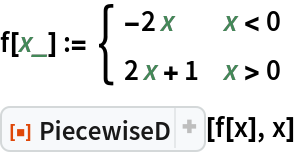Out=Compute the derivatives of a function whose domain is not an interval:

 In:=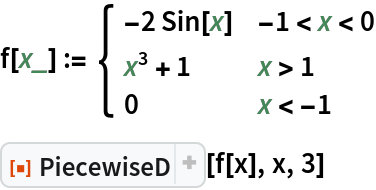Out=Compute just the third derivative:

 In:=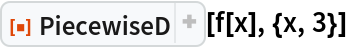Out=Plot the function together with its first three derivatives:

 In:=Out=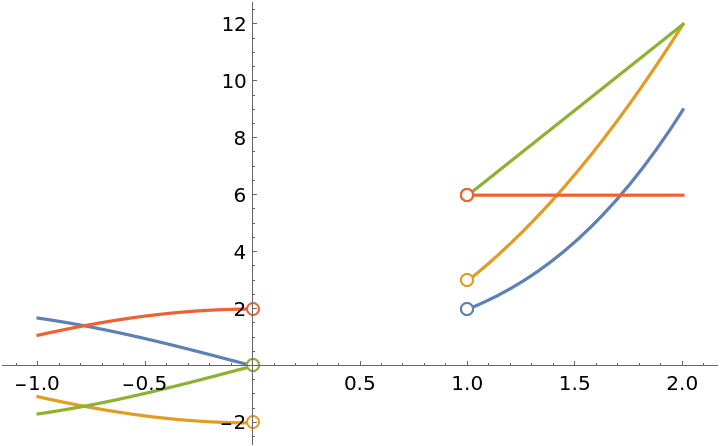### Scope (4)

Find and plot the first- and second-order derivatives. The function and its first-order derivative are continuous at x=0, but not the second-order derivative:

 In:=Out=Check that the first derivative is continuous:

 In:=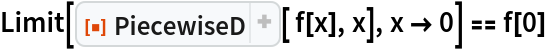Out=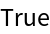Check that the second derivative is not continuous:

 In:=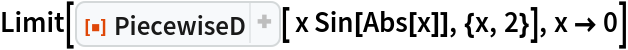Out=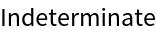Plot the results:

 In:=Out=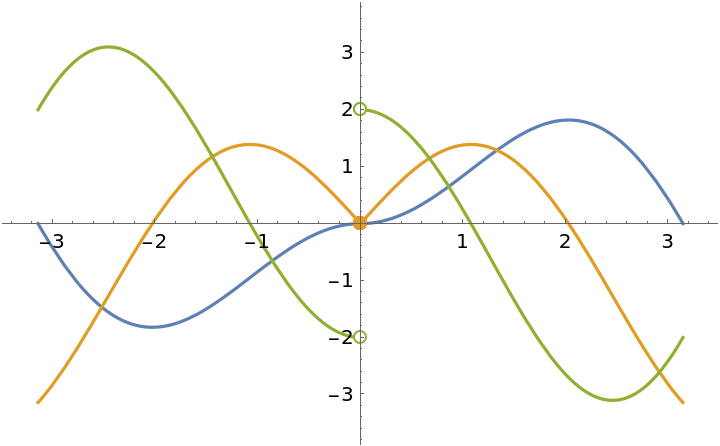### Properties and Relations (1)

Show the difference between PiecewiseD and D:

 In:=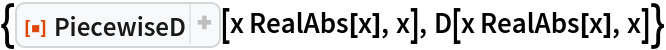Out=### Applications (5)

The following function has a removable discontinuity at x=3 and an infinite discontinuity at x=4:

 In:=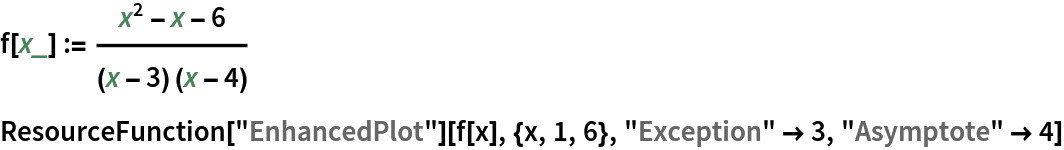Out=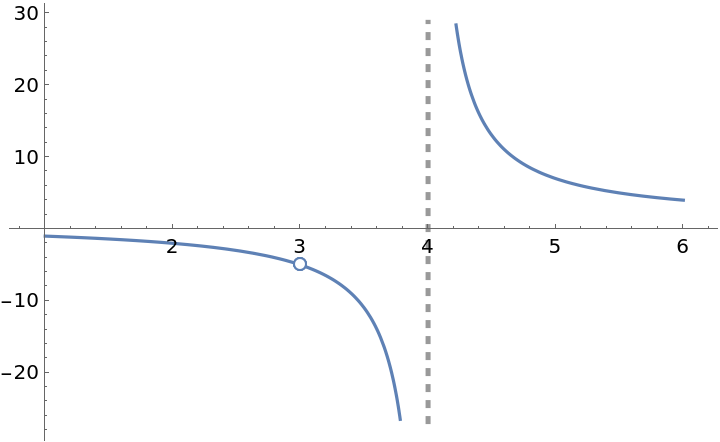Extend the definition at x=3 to make the extended function continuous there:

 In:=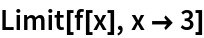Out=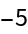In:=Out=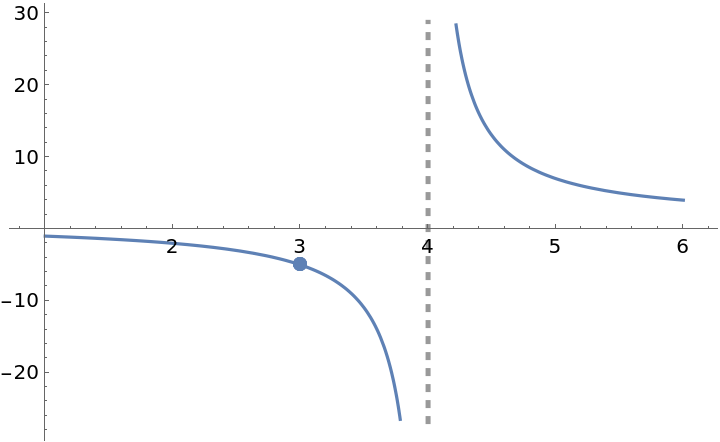The extended function is actually differentiable at x=3:

 In:=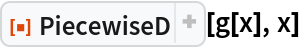Out=The resource function EnhancedPlot produces a correct plot:

 In:=Out=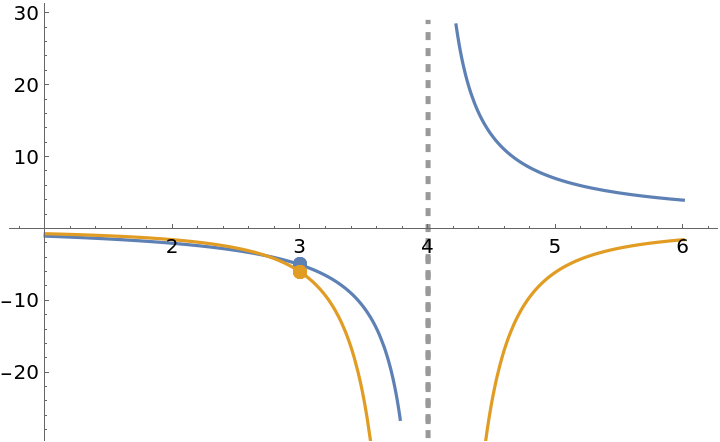The function g is differentiable at x=0 and PiecewiseD returns the correct value, 1. The function D, however, returns the value 0 for the derivative at x=0:

 In:=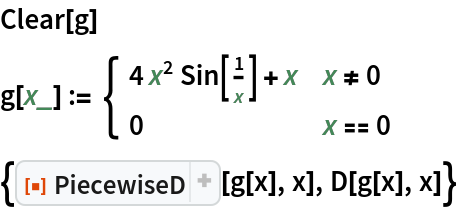Out=In:=Out=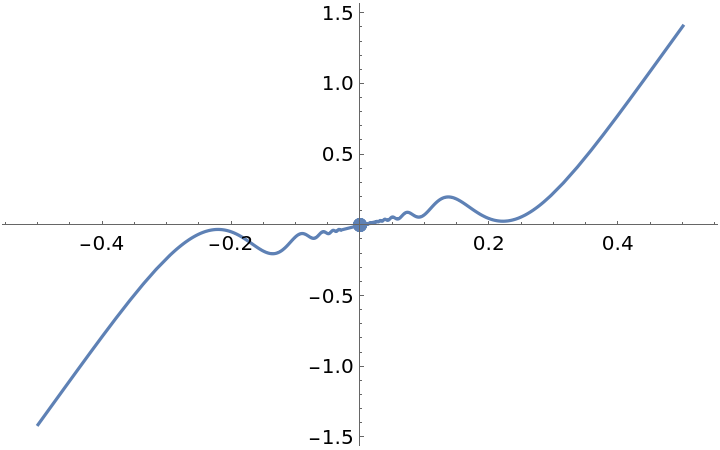However, the derivative is not continuous:

 In:=Out=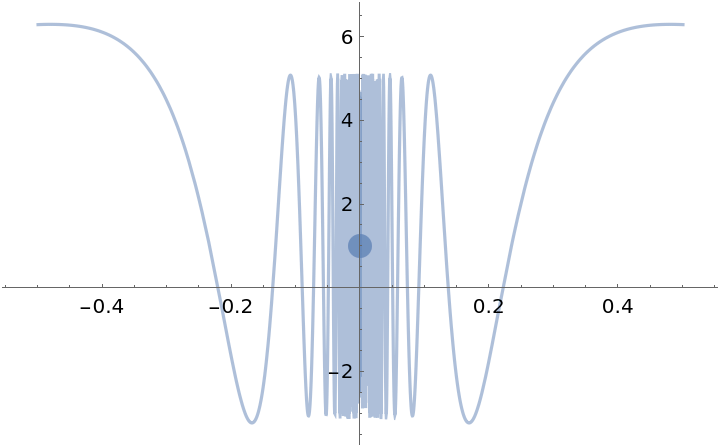This function is differentiable at x=0 and its derivative is continuous there:

 In:=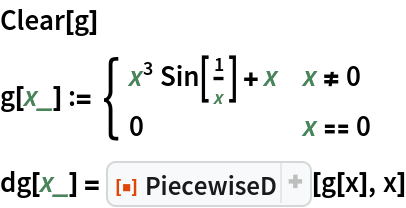Out=In:=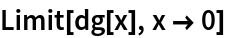Out=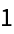Plot the result using the resource function EnhancedPlot:

 In:=Out=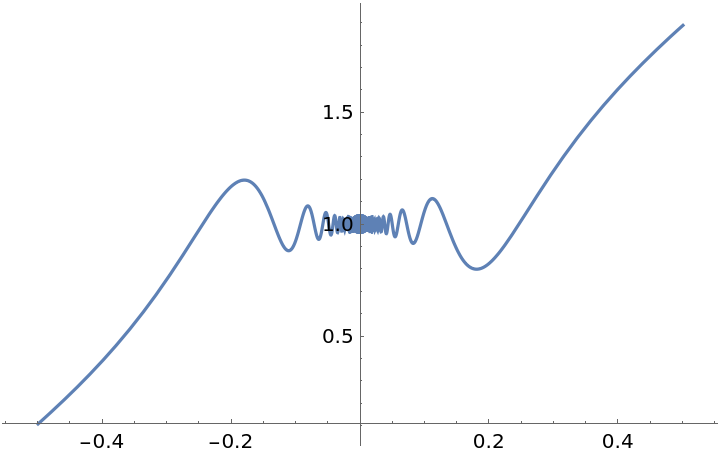A function with a singularity at x=-1 and x=1; PiecewiseD returns the correct result. Note that if this expression is simplified, the singularity at x=1 will be lost:

 In:=Out=The resource function EnhancedPlot is able to produce a correct plot:

 In:=Out=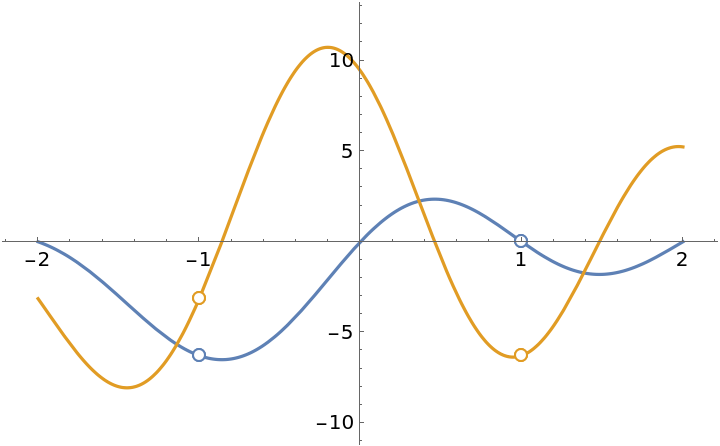Extend the function so that it becomes continuous at -1 and 1:

 In:=Out=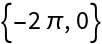In:=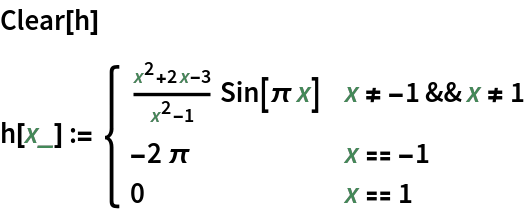The first and second derivatives are continuous at ±1:

 In:=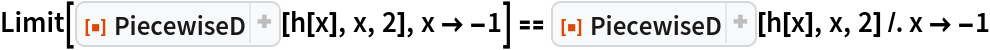Out=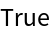In:=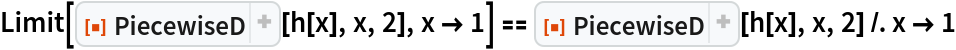Out=Plot the extended function and its first two derivatives:

 In:=Out=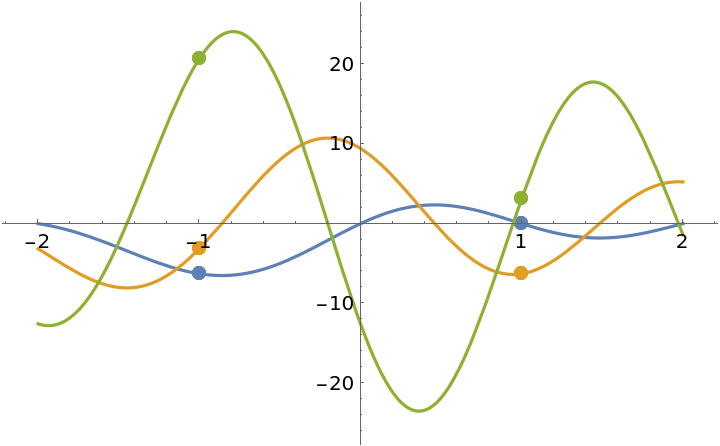A classic example of a nonzero infinitely differentiable function all of whose derivatives at x=0 are 0 and hence all of whose Taylor polynomials based at 0 are the zero polynomial:

 In:=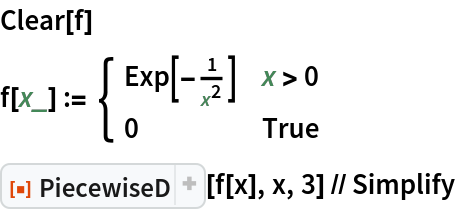Out=Illustrate with ResourceFunction["EnhancedPlot"]:

 In:=Out=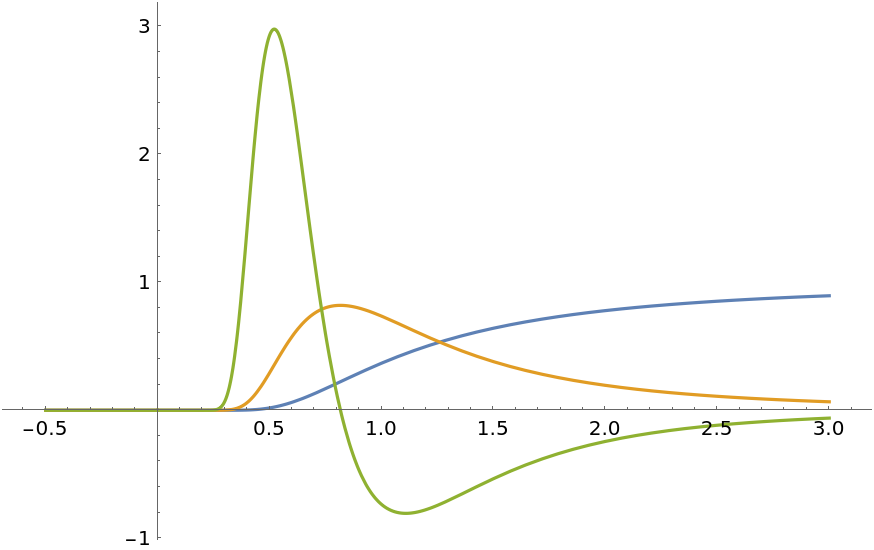## Publisher

Dennis M Schneider

## Version History

• 2.0.1 – 16 August 2022
• 2.0.0 – 09 August 2022
• 1.0.0 – 23 September 2020